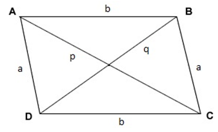# Diagonal of Parallelogram Formula

## Diagonal of Parallelogram Formula with Examples and Practice Questions

A parallelogram is a quadrilateral in which both pairs of opposite sides are parallel. Some of the properties of a parallelogram are that its opposite sides are equal, its opposite angles are equal and its diagonals bisect each other.
Let’s consider the following parallelogram whose sides are known, say $a$ and $b$. To find the length of one of its diagonals , we can use the cosine rule for triangles.In $\Delta ADC$, using the cosine rule, we calculate the length of $AC$ as follows.
$A{C^2} = A{D^2} + D{C^2} - 2 \times AD \times DC \times \cos \,\angle ADC \Rightarrow {p^2} = {a^2} + {b^2} - 2ab\cos \,\angle ADC$
Similarly in $\Delta ADB$we have the following equation.
$D{B^2} = A{D^2} + A{B^2} - 2 \times AD \times DB \times \cos \,\angle DAB \Rightarrow {q^2} = {a^2} + {b^2} - 2ab\cos \,\angle DAB$
We know that adjacent angles in a parallelogram are supplementary. So we have the following equations.
$\cos \angle DAB = \cos \pi - \angle ADC = - \cos \angle ADC$
So we have the following property.
${p^2} + {q^2} = {a^2} - {b^2} - 2ab\cos \angle ADC + {a^2} + {b^2} + 2ab\cos \angle ADC$
${p^2} + {q^2} = 2\left( {{a^2} + {b^2}} \right)$
Therefore, to calculate the diagonal lengths individually, we can use the following formulas.
$\begin{gathered} p = \sqrt {{a^2} + {b^2} - 2ab\cos \theta } \hfill \\ q = \sqrt {2\left( {{a^2} + {b^2}} \right) - {p^2}} \hfill \\ \end{gathered}$
Also note that the longer diagonal is opposite to the larger of the parallelogram’s angles, which is a direct result of the cosine rule.
In the case of a rectangle, which is a type of parallelogram in which the interior angles are 90°, the diagonal formula reduces to the following. The following result can also be obtained by applying the Pythagorean theorem to the rectangle.
$\begin{gathered} {p^2} = {q^2} = {l^2} + {b^2} \hfill \\ p = q = \sqrt {{l^2} + {b^2}} \hfill \\ \end{gathered}$
Let’s look at the following example to understand how to use these formulas.

Question:
If one of the angles of a parallelogram is 60° and its adjacent sides are 4 cm and 6 cm long, then evaluate the lengths of its diagonals.
Solution:
$a = 4\,cm,\,\,b = 6\,cm,\,\,\theta = {60^ \circ }$
$\begin{gathered} p = \sqrt {{a^2} + {b^2} - 2ab\cos \angle \theta } \hfill \\ \,\,\,\,\, = \sqrt {{4^2} + {6^2} - 2 \times 4 \times 6 \times \cos {{60}^ \circ }} \hfill \\ \,\,\,\,\, = \sqrt {16 + 52 - 48 \times \frac{1}{2}} = \sqrt {28} = 2\sqrt 7 \,cm \hfill \\ \end{gathered}$
$\begin{gathered} q = \sqrt {2\left( {{a^2} + {b^2}} \right)} \hfill \\ \,\,\,\, = \sqrt {2 \times \left( {{4^2} + {6^2}} \right) - {{\left( {2\sqrt 7 } \right)}^2}} \hfill \\ \,\,\,\, = \sqrt {104 - 28} = \sqrt {76} = 2\sqrt {19} \,cm \hfill \\ \end{gathered}$

Why don’t you try to solve the following problem to see if you have mastered these formulas?

Question:
One of the angles of a parallelogram is 135° and its diagonals are $3\,cm$ and $3\sqrt 5 \,cm$ respectively. Find the perimeter of the parallelogram.
Options:
(a) $4\left( {3 + \sqrt 2 } \right)\,cm$
(b) $3\left( {2 + \sqrt 2 } \right)\,cm$
(c) $12\,cm$
(d) $6\left( {1 + \sqrt 2 } \right)\,cm$
Solution:
$p = 3\,cm,\,\,q = 3\sqrt {5\,} \,cm,\,\,\theta = {135^ \circ }$
$\begin{gathered} \,\,\,\,\,{p^2} = {a^2} + {b^2} - 2ab\cos \theta \hfill \\ \Rightarrow {\left( {3\sqrt 5 } \right)^2} = {a^2} + {b^2} - 2ab\cos {135^ \circ } \hfill \\ \Rightarrow {a^2} + {b^2} - 2ab\left( { - \frac{1}{{\sqrt 2 }}} \right) = 45 \hfill \\ \Rightarrow {a^2} + {b^2} + \sqrt 2 ab = 45 \hfill \\ \end{gathered}$
$\begin{gathered} \,\,\,\,\,{p^2} + {q^2} = 2\left( {{a^2} + {b^2}} \right) \hfill \\ \Rightarrow {3^2} + {\left( {3\sqrt 5 } \right)^2} = 2\left( {{a^2} + {b^2}} \right) \hfill \\ \Rightarrow {a^2} + {b^2} = \frac{{9 + 45}}{2} = 27 \hfill \\ \end{gathered}$
Now, we can solve these two equations to calculate a and b.
$\begin{gathered} 27 + \sqrt 2 ab = 45 \hfill \\ \Rightarrow \sqrt 2 ab = 18 \Rightarrow ab = 9\sqrt 2 \Rightarrow b = \frac{{9\sqrt 2 }}{a} \hfill \\ \end{gathered}$
Substituting in one of the equations, we get the following.
$\begin{gathered} {a^2} + {\left( {\frac{{9\sqrt 2 }}{a}} \right)^2} = 27 \hfill \\ \Rightarrow {a^2} + \frac{{162}}{{{a^2}}} = 27 \hfill \\ \Rightarrow {a^4} - 27{a^2} + 162 = 0 \hfill \\ \end{gathered}$
Solving this quadratic and ignoring the negative answers (because lengths cannot be negative), we get the following values.
$a = 3\,cm,3\sqrt 2 \,cm$
For the corresponding values of a, we get values of b as follows.
$b = 3\,\sqrt 2 \,cm,3\,cm$
Therefore, the dimensions of the parallelogram are $3\,cm$ and $3\sqrt 2 \,cm$. Hence its perimeter is calculated as follows.
${\text{perimeter}} = 2\left( {a + b} \right) = 2\left( {3 + 3\sqrt 2 } \right) = 6\left( {1 + \sqrt 2 } \right)\,cm\,$